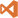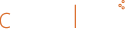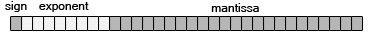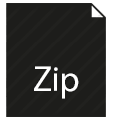HOME AUTHORMIXEDIT +ARTICLES +TUTORIALS# Article - Floating Point - Theory and Practice

## Introduction

Since I have been playing around with the floating point format these days I thought it was a good idea to write everything down for future reference. I want to thank Enrico Gullotti for reviewing this article.

Floating point is a common way to represent real numbers with the maximum amount of possible precision within the limited quantity of bits available. This approach is opposed to the fixed point notation, where, given N bits of precision, we dedicate N/2 bits for the integer part (123) and N/2 bits for the decimal part (321).

In the half of this article we will explore the fixed point notation to see its limits and get a grasp of the binary representation of real numbers. We will then introduce the floating point concept which describes how to distribute a fixed amount of bits to three components called sign, exponent and mantissa; we will then write a software implementation that construct 32 bits floating point numbers.

## Binary Real

As a first step let's see how we can represent the number 123.321 in binary assuming that we have 16 bits of space. The simplest way is to split the available bits between the integer part and the decimal part, so 8 bits each. This is what is called fixed point representation, (or fixed point notation). To create a binary number, in fixed point notation, from a number in the most familiar base ten, we need to apply two different algorithms to the integer and the decimal part of the value. Let's see the integer part first.

The integer part:

As a first step to the conversion we need to calculate what is the biggest representable number, and since we are using 8 bits we can represent an integer number between 0 and 255. The way to calculate the biggest number we can represent is done adding together a sequence of "two to the power of all the bits". For instance with 5 bits the biggest number we can represent is 31:

$2^0+2^1+2^2+2^3+2^4 = 1+2+4+8+16 = 31$

Notice how the number of elements we can actually represent is 32 because of the 0 element.

With 3 bits we can represent 8 numbers, and the biggest is $2^0+2^1+2^2=7$ (0,1,2,3,4,5,6,7).

The technique we are using to calculate the greatest representable number can be generalised to convert a generic sequence of bits to an integer number. Now, back to our case, the number we want to represent using 8 bits is 123; we can modify the formula we have seen, and for 8 bits numbers the formula reads as follow:

$b_0 2^0 + b_1 2^1 + b_2 2^2 + b_3 2^3 + b_4 2^4 + b_5 2^5 + b_6 2^6 + b_7 2^7 = Integer Number$

Where $b_i$ is the i-th bit in the sequence starting from right. So the binary number 00000111 can be converted to an integer like this $1 \cdot 2^0 + 1 \cdot 2^1 + 1 \cdot 2^2 + 0 \cdot 2^3 + 0 \cdot 2^4 + 0 \cdot 2^5 + 0 \cdot 2^6 + 0 \cdot 2^7 = 7$.

What we are really interested in is the opposite process, how to convert our number, 123, into it's decimal representation. The idea is to "reverse" the previous formula, and the following algorithm shows how to store the binary in a string:

std::string binaryRepresentation = "";
unsigned int intPart = 123;
for(unsigned int bitIndex = 0; bitIndex <= sizeof(unsigned int) * 8; ++bitIndex)
{
bool bitSet = (intPart % 2) == 1;
intPart = intPart / 2;
binaryRepresentation = std::string(bitSet ? "1" : "0") + binaryRepresentation;
}

The number 123 decimal is therefore equal to 01111011 in binary.

The decimal part:

The principles to convert the decimal part to binary are the same of the one presented, but with a few tweaks. First thing, the formula to calculate the decimal part is slightly different and for 8 bits it reads as follow:

$b_7 2^{-1} + b_6 2^{-2} + b_5 2^{-3} + b_4 2^{-4} + b_3 2^{-5} + b_2 2^{-6} + b_1 2^{-7} + b_0 2^{-8} = Decimal Number$

Please notice how the bits start from the seventh and go down to the zero-th. If we want to represent 0.5 we just need the seventh bit to be on so that we have $2^-1 = 0.5$, while if we want 0.625 we would need bits seven and five (2^-1 + 2^-3 = 0.625). It's the same idea we used for the integers but with fractions. If you imagine having only 2 bits of precision it's quite easy to see how small the amount of numbers that we can represent is:

 $0 * 2^{-1} + 0 * 2^{-2} = 0$ $1* 2^{-1} + 0 * 2^{-2} = 0.5$ $0 * 2^{-1} + 1 * 2^{-2} = 0.25$ $1 * 2^{-1} + 1 * 2^{-2} = 0.75$

As you would expect, with 2 bits we can represent 4 numbers, and using the previous formula the actual numbers are 0, 0.5, 0.75, 0.25.

If we reverse this formula we can convert a decimal number (stripped of its integer part) to its binary representation.

long long overflowContainer = decPart;
for(unsigned long long i = 0; i < numberOfDecimalDigits; ++i) overflowMask *= 10;
// Run through all the output bits we have, and for each one calculate its state
for(unsigned int bitIndex = 1; bitIndex <= sizeof(unsigned int)*8 && overflowContainer!=0; ++bitIndex)
{
overflowContainer *= 2;
{
*decPartBinaryOut |= 1 << (sizeof(unsigned int) * 8 - bitIndex);
}
}

You may have noticed that we read the decimal part as an integer; I have decided to do this is to avoid using any hardware floating point, mainly for educational purposes but also to avoid introducing the error of the floating point approximation.

Finally, the decimal part 0.321 is converted in binary to 0.01010010001011010000111001010110b.

Fixed point number:

If we run the two algorithms as follow:

// Convert integer parts into binary real parts
// E.g: "5.5" would return intPartBinaryOut = 101b = 5,
// decPartBinaryOut = 10000000000000000000000000000000b = 2147483648
void convertToBinaryReal(unsigned int intPart,
unsigned int decPart,
unsigned long long numberOfDecimalDigits,
unsigned int *intPartBinaryOut,
unsigned int *decPartBinaryOut) const
{
// The integer part is already in a form we like; no conversion is actually needed,
//just copy it across
*intPartBinaryOut = intPart;
// The decimal part needs to be converted
*decPartBinaryOut = 0;
long long overflowContainer = decPart;
for(unsigned long long i = 0; i < numberOfDecimalDigits; ++i) overflowMask *= 10;
// Run through all the output bits we have, and for each one calculate its state
for(unsigned int bitIndex = 1; bitIndex <= sizeof(unsigned int)*8 && overflowContainer!=0;++bitIndex)
{
overflowContainer *= 2;
{
*decPartBinaryOut |= 1 << (sizeof(unsigned int) * 8 - bitIndex);
}
}
}

We can finally convert any real number to its binary representation; our 123.321 can therefore be written as 1111011.01010010001011010000111001010110b.

## Floating Point

The main drawback of the fixed point method, is that it doesn't scale nicely, so if we have a lot o numbers with a small integer part (like 0.123321789) we would be wasting the top 8 bits to represent 0. If we know that we don't need much precision on either side (the integer part or the decimal part), why not to assign more bits to the side that needs them the most? That's the main idea behind floating point numbers. How to achieve it though? Well, with a couple of really clever usage of the available memory and number notations.

Floating point numbers are expressed in a notation very similar to the scientific one. The idea is that every number can be represented by the following formula:

$Floating Point = sign \cdot 2^{exp} \cdot 1.mantissa$

where $sign$ can be +1 or -1, $exp$ is an integer number (either positive or negative) and $mantissa$ is a binary number (you may find this formula elsewhere with 10 as a base for your exponent, in which case your mantissa has to be expressed as a number in base 10). So how do we represent a number such as 0.125 with this notation?

2. Convert the real number to binary
3. Shift it so that it has a single one in the integer part and take note of the shift amount
4. Encode the shift amount into the exp part of the float (left negative, right positive)
5. Encode the decimal part of the binary real into the mantissa

For 0.125 we have a positive sign, so we save that into the sign field. Then we convert 0.125 into binary real, which is 0.001b. We now need to shift the number so that it has 1 in the integer part, therefore we have a shift to the left of 3 positions. At this point we have the following $2^{-3} \cdot 1.0b = 0.001b$. So the exponent is -3, and the mantissa is 0. Let's see the code for the conversion.

Floating Point 32 bits:

On an actual machine you will need to define a certain amount of bits to be assigned to sign, exponent and mantissa. Sign is really a binary state, so it's always assigned a single bit. In the standardized format (IEEE 754) for a 32 bits float the exponent is assigned 8 bits and the mantissa 23 bits.IEEE 754 Single Precision Format

It's also important to notice that in the current IEEE specification (which is what defines what is implemented in your hardware) the exponent is not saved in 2 complement but with an implicit bias. This means that when you read the value in memory you then need to subtract a bias value (127 in case of 8 bits) to obtain the actual exponent. Similarly, if you want to encode an exponent you will have to add the bias first. For example, take 1.0 represented as a float 32. If you check what is in memory you will find the following bits: , which means sign bit to 0 (positive), exponent is 01111111b = 127 and mantissa = 0. If you subtract 127 to the exponent you get 0, and if you then plug all the values in the formula you get 1.0 as expected.

// Convert binary real parts into exponent and mantissa
// E.g: intPartBinary = 101b = 5, decPartBinary = 10000000000000000000000000000000b = 2147483648 generate an output of *exponentOut = 129 and *mantissaOut = 1610612736
void convertBinaryRealToFloat(unsigned int intPartBinary,
unsigned int decPartBinary,
unsigned char *exponentOut,
unsigned int *mantissaOut ) const
{
int uncompressedExponent = 0;
// If the integer part is 0 we will get a negative exponent
if(intPartBinary == 0)
{
// 0.0b is a special case
if(decPartBinary == 0)
{
uncompressedExponent = -127;
*mantissaOut = 0;
}
else
{
uncompressedExponent = 32 - findLeftmostBitSet(decPartBinary);
*mantissaOut = decPartBinary << uncompressedExponent;
uncompressedExponent = -uncompressedExponent;
}
}
// else we get a positive exponent
else
{
uncompressedExponent = findLeftmostBitSet(intPartBinary);
*mantissaOut = decPartBinary >> uncompressedExponent;
*mantissaOut = *mantissaOut | (intPartBinary << (32 - uncompressedExponent));
}
*exponentOut = uncompressedExponent + 127;
}

Floating Point 16 bits and 64 bits:

Floating point at 64 bits are supported by many languages and are usually known as double, while 16 bits floating point are more rare and are often referred to as half. They work in exactly the same way of their 32 bits counterpart but they reserve more or less bits for each component. Also the exponent bias changes as a consequence.

Special cases:

Some combinations of values for exponent and mantissa are reserved to represent special cases like +infinite, - infinite, NaN (not a number) and zero (how can we represent zero if we always have an implied 1 in the formula! You need a special case). These cases are all defined in the standard IEEE 754. The specification states that you can represent infinite by filling the exponent with ones (in binary), so with 8 bits it will be 255. You can still use the sign bit to make it positive or negative. If you set all the components to zero you get the special case for 0. You can still use the sign bit to represent positive or negative zeroes. Indefinite values (NaN) have all ones in the exponent and a non zero value for the mantissa (often all zeros apart from the most significant bit).Floating Point Visual Studio 2010 project file and source code is provided Download sources (zip ~4 Kb)